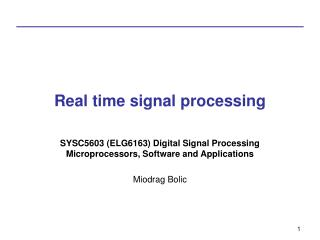DownloadDownload PresentationReal time signal processing

# Real time signal processing

Télécharger la présentation## Real time signal processing

- - - - - - - - - - - - - - - - - - - - - - - - - - - E N D - - - - - - - - - - - - - - - - - - - - - - - - - - -
##### Presentation Transcript

1. Real time signal processing SYSC5603 (ELG6163) Digital Signal Processing Microprocessors, Software and Applications Miodrag Bolic

2. Outline • Real time signal processing • Structural levels of processing • Properties and parameters of signal processing algorithms • Definitions of throughput, latency, concurrency, … In order to prepare this material, Chapters 1 and 3 from [Ackenhusen99 ] are used. 2

3. DSP System

4. Real time signal processing • x(n) input discrete time signal representation sampled every Tx. • y(n) input discrete time signal representation sampled every Ty. • Signal processing is a transformation F of input samples x(n) to obtain the output signal y(m)=F(x(n)) • Tc is a computation time needed to process L input samples. • System that has Tc≤LTx is said to operate in real-time.

5. Real time signal processing - Conditions • Conditions for real-time processing • the input sample period Tx • the complexity of the transformation F • the speed of the computer(s) which compute F(x(n)) as measured by Tc

6. Non-real time signal processing

7. Structural levels of processing • Stream processing • all computations with one input sample are completed before the next input sample arrives • Block processing • each input sample x(n) is stored in memory before any processing occurs upon it. After L input samples have arrived, the entire collection of samples is processed at once. • Vector processing • systems with several input and/or output signals being computed at once: can work with streams or blocks

8. Stream processing

9. Block processing • Short-time stationarity of signals • Advantages • Efficiency: • Fast algorithms such as FFT can be applied • Some algorithms (median) require access to all the samples in the block and are difficult to execute in a stream manner. • Disadvantages • Latency

10. Parameters of algorithms related to complexity • Throughput; • Range and precision of numbers; • Data-dependent execution, whereby the instruction sequence is influenced by the incoming data; • Precedence relations within the algorithm, as well as the lifetime of data values within the computation; • Global versus local communication of data; • Random versus regular sequencing of data addresses; • Diversity of operations and the amount of "difficult" instructions

11. Timing parameters • The critical path determines the time it takes to complete an iteration of the computation. • The latency of an algorithm is the time it takes to generate an output value from the corresponding input value.

12. Throughput • Throughput is defined as the reciprocal of the time deference between successive outputs. • It depends on: • number of operations, Examples: Speech coding ~ 100’s of operation per sample Video applications ~5 to 10 operations per sample • amount of data to process, and • time available to process

13. Range and precision of numbers • A number is represented with a fixed number of bits - tradeoff between dynamic range and precision. • Dynamic range is the range between the most negative and the most positive number encountered. • The number of bits determines the number of numeric levels available • Complexity increases with the number of bits. • In a purpose-built (custom) architecture, increasing the number of bits increases the area, approximately as the square of the number of bits.

14. Data-dependent execution • High-speed computing is most easily achieved for algorithms that are regular, i.e., that perform the same operations on each piece of data. • Data-dependent computations and data precedence requirements for sequential execution pose obstacles to achieving task parallelism (executing multiple tasks in parallel). • The requirement of global communication increases the difficulty of achieving data parallelism (performing parallel computations on subsets of the data). • Data dependencies are studied through temporal and spatial locality: • Temporal locality is described as the tendency for a program to reuse the data or instructions which have recently been used. • Spatial locality is the tendency for a program to use the data or instructions neighboring those which were recently used.

15. Data lifetime • Computations that use a piece of data once and then discard it are more amenable to stream processing algorithms • Stream processing algorithms require less storage, avoid the need to again find a piece of data from within a random memory array, and reduce the latency of results. • Block processing algorithms, which collect all samples at once before acting upon them, require time to accumulate numbers, which introduces latency.